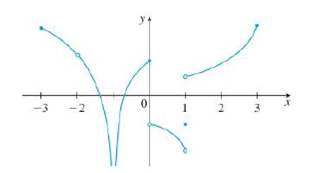Chapter 2.5, Problem 4E### Single Variable Calculus: Early Tr...

8th Edition
James Stewart
ISBN: 9781305270343

#### Solutions

Chapter
Section### Single Variable Calculus: Early Tr...

8th Edition
James Stewart
ISBN: 9781305270343
Textbook Problem

# From the graph of g, state the intervals on which g is continuous.To determine

To state: The intervals on which g is continuous from the graph of g.

Explanation

Definition used:

1. A function f is continuous at a number a if limxaf(x)=f(a).

2. A function f is continuous on an interval if it is at continuous every number in the interval.

Calculation:

The graph of g is defined at the right endpoint of −3 and it has a break at −2. But the graph has no hole at every number on the interval [3,2).

Therefore, by definition 2, the function g is continuous on the interval [3,2). That is, g is continuous from the right at −3.

The graph of g is undefined at the right endpoint of −2 and the left endpoint of −1. But it has no hole at every number on the interval (2,1).

Therefore, by definition 2, the function g is continuous on the interval (2,1).

The graph of g is undefined at the right endpoint of −1 and defined at the left endpoint of 0. The graph has no hole at every number on the interval (1,0].

Therefore, by definition 2, the graph of g is continuous on the interval (1,0]

### Still sussing out bartleby?

Check out a sample textbook solution.

See a sample solution

#### The Solution to Your Study Problems

Bartleby provides explanations to thousands of textbook problems written by our experts, many with advanced degrees!

Get Started